## 信息熵的时间度量模型muti

R的极客理想系列文章，涵盖了R的思想，使用，工具，创新等的一系列要点，以我个人的学习和体验去诠释R的强大。

R语言作为统计学一门语言，一直在小众领域闪耀着光芒。直到大数据的爆发，R语言变成了一门炙手可热的数据分析的利器。随着越来越多的工程背景的人的加入，R语言的社区在迅速扩大成长。现在已不仅仅是统计领域，教育，银行，电商，互联网….都在使用R语言。

• 张丹(Conan), 程序员/Quant: Java,R,Nodejs
• blog: http://blog.fens.me
• email: bsspirit@gmail.com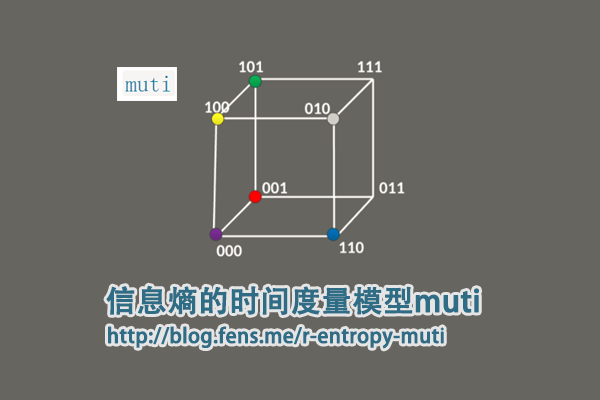1. muti包介绍
2. muti包函数定义
3. muti包使用
4. 实战案例

## 1. muti包介绍

muti包是用于计算两个离散随机变量之间的互信息(MI)的R包。 muti包设计目标，是考虑时间序列分析的情况下开发的，但没有任何方法可以将方法与时间索引本身联系起来。

muti项目的主页：https://mdscheuerell.github.io/muti/

muti包中用来计算互信息的计算过程，包括以下几步：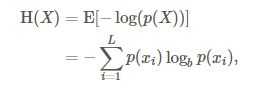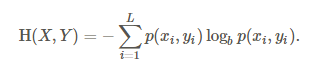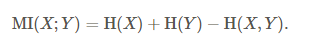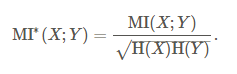• Win10 64bit
• R: 3.4.2 x86_64-w64-mingw32/x64 b4bit

muti目前还没有发布到CRAN上面，需要通过devtools包从github上进行安装。安装操作如下。

``````
> if(!require("devtools")) {
>  install.packages("devtools")
>  library("devtools")
> }
> devtools::install_github("mdscheuerell/muti")
``````

## 2. muti包函数定义

muti包，有很强的专业性，没有太多的函数定义，函数都是为了实现模型的算法。

• muti()，主函数，用于计算两个向量的互信息并画图输出
• mutual_info()，计算互信息
• newZ()，基于原始数据的重新采样创建新矢量。
• symbolize()，将数字向量转换为符号向量。
• transM()，估计用于计算互信息上的临界阈值的转换矩阵。
• plotMI()，画图输出

2.1 muti()函数：

``````
muti(x, y, sym = TRUE, n_bins = NULL, normal = FALSE, lags = seq(-4, 4), mc = 100, alpha = 0.05)
``````

• x, 向量
• y, 向量
• sym，是否转换成符号向量，默认TRUE,
• n_bins, 离散化方法，当sym=FALSE时， n_bins = ceiling（2 * length(x)^(1/3))
• normal, 是否标准化，默认FALSE
• lags, 滞后周期，默认为seq(-4, 4)
• mc, 用于估计互信息的临界阈值的蒙特卡洛数量的模拟，默认100,
• alpha, 用于定义临界阈值的取值点0.05

2.2 mutual_info()函数：

``````
# 源函数
mutual_info <- function(su,normal) {
## function to calculate mutual information between 2 time series
## need to remove obs with NA's b/c they don't contribute to info
su <- su[!apply(apply(su,1,is.na),2,any),]
## get number of bins
bb <- max(su)
## get ts length
TT <- dim(su)
## init entropies
H_s <- H_u <- H_su <- 0
for(i in 1:bb) {
## calculate entropy for 1st series
p_s <- sum(su[,1] == i)/TT
if(p_s != 0) { H_s <- H_s - p_s*log(p_s, 2) }
## calculate entropy for 2nd series
p_u <- sum(su[,2] == i)/TT
if(p_u != 0) { H_u <- H_u - p_u*log(p_u, 2) }
for(j in 1:bb) {
## calculate joint entropy
p_su <- sum(su[,1]==i & su[,2]==j)/TT
if(p_su != 0) { H_su <- H_su - p_su*log(p_su, 2) }
} ## end j loop
} ## end i loop
## calc mutual info
MI <- H_s + H_u - H_su
if(!normal) { return(MI) } else { return(MI/sqrt(H_s*H_u)) }
}

# 修改后的函数
library(philentropy)
mutual_info <- function(su,normal){
n<-nrow(su)
px<-table(su[,1])/n
py<-table(su[,2])/n

hX<-H(px)
hY<-H(py)

tf<-table(su[,1],su[,2])
pXY<-prop.table(tf)
hXY<-JE(pXY)

MI<-hX+hY-hXY
if(normal){
return(MI/sqrt(hX * hY))
}else{
return(MI)
}
}
``````

2.3 symbolize()函数：

``````
# 源函数
symbolize <- function(xy) {
## of interest and converts them from numeric to symbolic.
## check input for errors
if(dim(xy) != 2) {
stop("xy must be an [n x 2] matrix \n\n", call.=FALSE)
}
## get ts length
TT <- dim(xy)
## init su matrix
su <- matrix(NA, TT, 2)
## convert to symbols
## loop over 2 vars
for(i in 1:2) {
for(t in 2:(TT-1)) {
## if xy NA, also assign NA to su
if(any(is.na(xy[(t-1):(t+1),i]))) { su[t,i] <- NA }
## else get correct symbol
else {
if(xy[t,i] == xy[t-1,i] & xy[t,i] == xy[t+1,i]) {
## same
su[t,i] <- 3
}
if(xy[t,i] > xy[t-1,i]) {
## peak
if(xy[t,i] > xy[t+1,i]) { su[t,i] <- 5 }
## increase
else { su[t,i] <- 4 }
}
if(xy[t,i] < xy[t-1,i]) {
## trough
if(xy[t,i] < xy[t+1,i]) { su[t,i] <- 1 }
## decrease
else { su[t,i] <- 2 }
}
} ## end else
} ## end t loop
} ## end i loop
## return su matrix
return(su)
}

# 优化后的函数
symbolize<-function(xy){
if(ncol(xy)!=2) {
stop("xy must be an [n x 2] matrix \n\n", call. = FALSE)
}

idx<-2:(nrow(xy)-1)
apply(xy,2,function(row){
na<-which(is.na(row[idx]+row[idx-1]+row[idx+1]))
n3<-which(row[idx]==row[idx-1] & row[idx]==row[idx+1])
n5<-which(row[idx]>row[idx-1] & row[idx]>row[idx+1])
n4<-which(row[idx]>row[idx-1] & row[idx]<=row[idx+1])
n1<-which(row[idx]<row[idx-1] & row[idx]<row[idx+1])
n2<-which(row[idx]<row[idx-1] & row[idx]>=row[idx+1])

line<-rep(0,length(idx))
line[na]<-NA
line[n1]<-1
line[n2]<-2
line[n3]<-3
line[n4]<-4
line[n5]<-5
c(NA,line,NA)
})
}
``````

2.4 transM()函数：

``````
# 源函数
transM <- function(x,n_bins) {
## helper function for estimating transition matrix used in
## creating resampled ts for the CI on mutual info
## replace NA with runif()
x[is.na(x)] <- runif(length(x[is.na(x)]),min(x,na.rm=TRUE),max(x,na.rm=TRUE))
## length of ts
tt <- length(x)
## get bins via slightly extended range
bins <- seq(min(x)-0.001,max(x),length.out=n_bins+1)
## discretize ts
hin <- vector("numeric",tt)
for(b in 1:n_bins) {
hin[x > bins[b] & x <= bins[b+1]] <- b
}
## matrix of raw-x & discrete-x
xn <- cbind(x,hin)
## transition matrix from bin-i to bin-j
MM <- matrix(0,n_bins,n_bins)
for(i in 1:n_bins) {
for(j in 1:n_bins) {
MM[i,j] <- sum(hin[-tt]==i & hin[-1]==j)
}
if(sum(MM[i,])>0) { MM[i,] <- MM[i,]/sum(MM[i,]) }
else { MM[i,] <- 1/n_bins }
}
return(list(xn=xn,MM=MM))
} ## end function

# 优化后的函数
transM <- function(x,n_bins){
x[is.na(x)] <- runif(length(x[is.na(x)]), min(x, na.rm = TRUE),max(x, na.rm = TRUE))
n<-length(x)
xn<-cbind(x,hin=cut(x,7))

hin<-xn[,2]
a<-data.frame(id=1:(n-1),a=hin[1:(n-1)])
b<-data.frame(id=1:(n-1),b=hin[2:n])
ab<-merge(a,b,by="id",all=TRUE)
ab\$v<-1
abm<-aggregate(v~a+b,data=ab,sum)

MM <- matrix(0, n_bins, n_bins)
apply(abm,1,function(row){
MM[row,row]<<-row
invisible()
})

MN<-apply(MM,1,function(row){
s<-sum(row)
if(s>0){
row/s
}else{
rep(1/n_bins,n_bins)
}
})
MN<-t(MN)
return(list(xn = xn, MM = MN))
}
``````

## 3. muti包使用

3.1 数据的输入和输出
muti()函数，是muti包的主函数，调用muti()就完成了这个包的全部功能。

• 输入，要求是2个数字型向量。
• 输出，包括了2个部分，1.数据计算结果，2.可视化的结果。

``````> set.seed(123)
> TT <- 30

# 创建两个数字型向量
> x1 <- rnorm(TT)
> y1 <- x1 + rnorm(TT)

# 计算互信息
> muti(x1, y1)
lag MI_xy MI_tv
1  -4 0.238 0.595
2  -3 0.473 0.532
3  -2 0.420 0.577
4  -1 0.545 0.543
5   0 0.712 0.584
6   1 0.393 0.602
7   2 0.096 0.546
8   3 0.206 0.573
9   4 0.403 0.625
``````

• lag: 滞后期，当lag=-1，length(x)=length(y)=26时，x取值[1:25],y取值[2:26]；当lag=1，length(x)=length(y)=26时，x取值[2:26],y取值[1:25]；
• MI_xy: 互信息
• MI_tv: 相应显着性阈值，用蒙特卡洛方法生成互信息，按从大到小排序后取1-alpha位置的值。
• 上图，原始数据的x,y两个变量
• 中图，符号转型后的x,y两个变量，把原始数据离散化为5个值，差异化更大
• 下图，滞后期的互信息（实线）和相应显着性阈值（虚线），同数据计算结果的输出。

3.2 离散化处理

muti包提供了2种离散化。

• 符号化： 把连续型的数据，映射到5个值的上，分别对应peak(峰值), increasing(增加), same(没变)，decreasing(减少)，trough(低谷)。比如，向量c(1.1,2.1,3.3,1.2,3.1)，不计算第一个值和最后一个值，第二个值2.1>第一个值1.1，第二个值2.1<第三个值3.3时，第二个值为增加。第2，3，4值，分别为c(“增加”，“峰值”，“低谷”)。
• 分箱法： 等宽分箱，如果没有指定箱数，则根据莱斯规则计算，其中n = ceiling(2*length(x)^(1/3))。

## 4. 实战案例

``````// 省略部分代码
date X601398 X000001
1  2014-10-21    2.92 2339.66
2  2014-10-22    2.91 2326.55
3  2014-10-23    2.92 2302.42
4  2014-10-24    2.92 2302.28
5  2014-10-27    2.90 2290.44
6  2014-10-28    2.92 2337.87
7  2014-10-29    2.94 2373.03
8  2014-10-30    2.98 2391.08
9  2014-10-31    3.04 2420.18
10 2014-11-03    3.01 2430.03
11 2014-11-04    3.02 2430.68
12 2014-11-05    2.97 2419.25
13 2014-11-06    2.97 2425.86
14 2014-11-07    2.97 2418.17
15 2014-11-10    3.05 2473.67
16 2014-11-11    3.16 2469.67
17 2014-11-12    3.12 2494.48
18 2014-11-13    3.12 2485.61
19 2014-11-14    3.14 2478.82
20 2014-11-17    3.11 2474.01
``````

3列数据，第一列是时间列，第二列是X601398人收盘价，第三列是X000001收盘价。从价格上看，2个数据集并不在同一个度量内，我们转化度量单位为每日收益率。

``````> head(ret,20)
X601398       X000001
2014-10-21           NA            NA
2014-10-22 -0.003424658 -5.603378e-03
2014-10-23  0.003436426 -1.037158e-02
2014-10-24  0.000000000 -6.080559e-05
2014-10-27 -0.006849315 -5.142728e-03
2014-10-28  0.006896552  2.070781e-02
2014-10-29  0.006849315  1.503933e-02
2014-10-30  0.013605442  7.606309e-03
2014-10-31  0.020134228  1.217023e-02
2014-11-03 -0.009868421  4.069945e-03
2014-11-04  0.003322259  2.674864e-04
2014-11-05 -0.016556291 -4.702388e-03
2014-11-06  0.000000000  2.732252e-03
2014-11-07  0.000000000 -3.170010e-03
2014-11-10  0.026936027  2.295124e-02
2014-11-11  0.036065574 -1.617031e-03
2014-11-12 -0.012658228  1.004588e-02
2014-11-13  0.000000000 -3.555851e-03
2014-11-14  0.006410256 -2.731724e-03
2014-11-17 -0.009554140 -1.940439e-03
``````

``````> head(rdf,20)
X601398       X000001
2014-10-22 -0.003424658 -0.0056033783
2014-10-23  0.000000000 -0.0159168426
2014-10-24  0.000000000 -0.0159766804
2014-10-27 -0.006849315 -0.0210372447
2014-10-28  0.000000000 -0.0007650684
2014-10-29  0.006849315  0.0142627561
2014-10-30  0.020547945  0.0219775523
2014-10-31  0.041095890  0.0344152569
2014-11-03  0.030821918  0.0386252703
2014-11-04  0.034246575  0.0389030885
2014-11-05  0.017123288  0.0340177633
2014-11-06  0.017123288  0.0368429601
2014-11-07  0.017123288  0.0335561577
2014-11-10  0.044520548  0.0572775531
2014-11-11  0.082191781  0.0555679030
2014-11-12  0.068493151  0.0661720079
2014-11-13  0.068493151  0.0623808588
2014-11-14  0.075342466  0.0594787277
2014-11-17  0.065068493  0.0574228734
2014-11-18  0.058219178  0.0498833164
``````

``````
> x<-rdf\$X601398
> y<-rdf\$X000001
> result<-muti(x,y)
> result
lag MI_xy MI_tv
1  -4 0.148 0.534
2  -3 0.158 0.464
3  -2 0.190 0.385
4  -1 0.351 0.425
5   0 0.646 0.398
6   1 0.528 0.340
7   2 0.262 0.461
8   3 0.207 0.437
9   4 0.260 0.518
``````
• 上图，为原始数据的x(上证综指)和y(工商银行)的累积收益率曲线。
• 中图，为符号转型后的x(上证综指)和y(工商银行)的累积收益率曲线。
• 下图，符号转型后的数据，滞后期从-4到4的9个的互信息（实线）和和相应显着性阈值（虚线）。

``````// 省略部分代码
> rsdf
lag MI_xy MI_tv       sd
1  -4 0.148 0.534 1.991886
2  -3 0.158 0.464 2.400721
3  -2 0.190 0.385 2.107189
4  -1 0.351 0.425 2.378768
5   0 0.646 0.398 1.477030
6   1 0.528 0.340 2.342482
7   2 0.262 0.461 1.997676
8   3 0.207 0.437 2.267211
9   4 0.260 0.518 2.116934
``````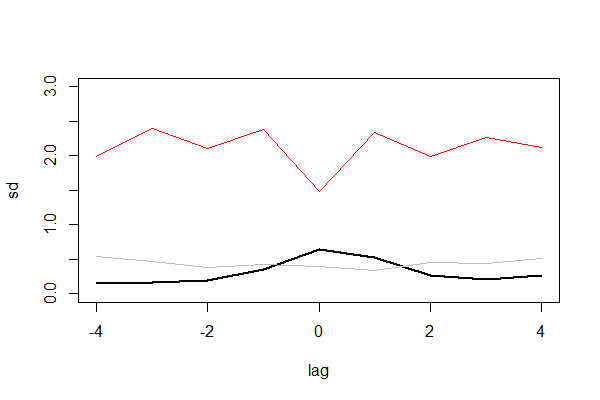This entry was posted in R语言实践